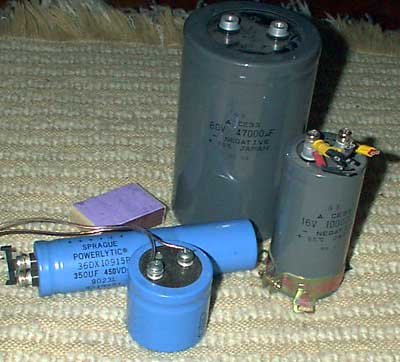# Very Big capacitors, few words

There are capacitors and there are very big capacitors. The last ones are with heavy duty terminal connectors for high current connections, and the capacity of them are quite big. Something like 47000mkf x 80V or 100000mkf (0.1F)x 16V. Such devices can store lots of energy. They are used, when high currents are used in pulses- like large printers, high power audio amplifiers. That 0.1F cap is very old, I think about 20 years old, but still holding the charge. I charged it to 14V, and after about 2 hours, my voltmeters shows 13V (after night it is still 12.5V!).

The energy stored in capacitor is calculated in Joules:

E = CU`2`/2 ; E in J, C in F, U in V.

So that cap can hold 27.6J of energy. As you see, the voltage is more important in energy calculation than capacitance. So another big cap- 350mkF x 450V can hold – ~73J of energy. And it is lighter than low voltage one. Ant this 73J can kill you.

The capacitance (C) is the amount of charge per volt of potential that a capacitor holds.

C =Q/U ; where Q = coulombs (the unit of charge) and U = Volts.

The average power in watts is:

P`av` = CU`2`/2t ; where t = time in seconds.

Using caps in pulse mode, the current is important. The ripple current is sometimes rated for a capacitor in RMS current. Remembering that

P = I`2`R ; where R in this case is ESR

It is plain to see, that this is a power dispassion rating. ESR is the sum of in-phase AC resistance. It includes resistance of the dielectric, plate material, electrolytic solution, and terminal leads at a particular frequency. ESR acts like a resistor in series with a capacitor (thus the name Equivalent Series Resistance). This resister can cause circuits to fail that look just fine on paper and is often the failure mode of capacitors.

How to measure the capacitance of such big caps? The formula is here:

`Uc = Usupply(1-2.718-t/(RC))`.

Discharge your cap by shortening the terminals, connect your cap to powerful and stabilised power supply in series with resistor, charge the cap for few seconds and measure the voltage of the capacitor. And compare to theoretical values:

` function program_r_c_time_voltage() {power_supply_voltage = eval(document.rc_time_to_voltage_calc.power_supply_voltage.value); capacitor_value_farads = eval(document.rc_time_to_voltage_calc.capacitor_value_farads.value); resistor_value_ohms = eval(document.rc_time_to_voltage_calc.resistor_value_ohms.value); charging_time_total = eval(document.rc_time_to_voltage_calc.charging_time_total.value); one_time_constant = eval(document.rc_time_to_voltage_calc.one_time_constant.value); capacitor_inst_voltage = eval(document.rc_time_to_voltage_calc.capacitor_inst_voltage.value); document.rc_time_to_voltage_calc.one_time_constant.value = eval(eval(document.rc_time_to_voltage_calc.capacitor_value_farads.value)*document.rc_time_to_voltage_calc.resistor_value_ohms.value); document.rc_time_to_voltage_calc.capacitor_inst_voltage.value = eval(document.rc_time_to_voltage_calc.power_supply_voltage.value*(1-Math.pow(2.718,(-document.rc_time_to_voltage_calc.charging_time_total.value/document.rc_time_to_voltage_calc.one_time_constant.value)))); document.rc_time_to_voltage_calc.charging_time_total.value = eval(Math.round(document.rc_time_to_voltage_calc.charging_time_total.value*1000000)/1000000); document.rc_time_to_voltage_calc.one_time_constant.value = eval(Math.round(document.rc_time_to_voltage_calc.one_time_constant.value*1000000)/1000000); document.rc_time_to_voltage_calc.capacitor_inst_voltage.value = eval(Math.round(document.rc_time_to_voltage_calc.capacitor_inst_voltage.value*1000000)/1000000);}`

``` Input Power Supply Voltage? Volts Capacitor Value? Farads Resistor Value? Ohms Total Charging Time? Seconds Output One Time Constant? Seconds Instantaneous Capacitor Voltage? Volts ```1.Administrator says:
2.Administrator says: